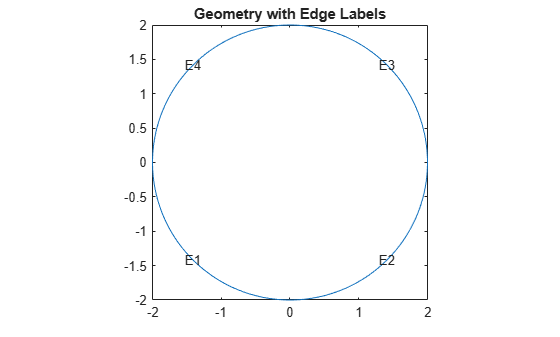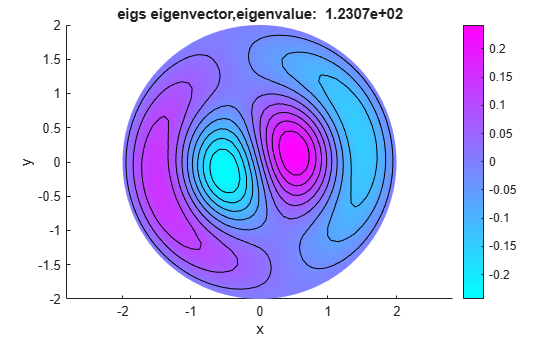# Vibration of Circular Membrane

This example shows how to calculate the vibration modes of a circular membrane.

The calculation of vibration modes requires the solution of the eigenvalue partial differential equation. This example compares the solution obtained by using the `solvepdeeig` solver from Partial Differential Toolbox™ and the `eigs` solver from MATLAB®. Eigenvalues calculated by `solvepdeeig` and `eigs` are practically identical, but in some cases one solver is more convenient than the other. For example, `eigs` is more convenient when calculating a specified number of eigenvalues in the vicinity of a particular target value. While `solvepdeeig` requires that a lower and upper bound surrounding this target, `eigs` requires only the target eigenvalue and the desired number of eigenvalues.

Create a PDE model.

`model = createpde;`

Create the circle geometry and include it in the model.

```radius = 2; g = decsg([1 0 0 radius]','C1',('C1')'); geometryFromEdges(model,g);```

Plot the geometry with the edge labels.

```figure pdegplot(model,"EdgeLabels","on") axis equal title("Geometry with Edge Labels")```Specify the coefficients.

```c = 1e2; a = 0; f = 0; d = 10; specifyCoefficients(model,"m",0,"d",d,"c",c,"a",a,"f",f);```

Specify that the solution is zero at all four outer edges of the circle.

`bOuter = applyBoundaryCondition(model,"dirichlet","Edge",(1:4),"u",0);`

Generate a mesh.

`generateMesh(model,"Hmax",0.2);`

Use `assembleFEMatrices` to calculate the global finite element mass and stiffness matrices with boundary conditions imposed using the nullspace approach.

```FEMatrices = assembleFEMatrices(model,"nullspace"); K = FEMatrices.Kc; B = FEMatrices.B; M = FEMatrices.M;```

Solve the eigenvalue problem by using the `eigs` function.

```sigma = 1e2; numberEigenvalues = 5; [eigenvectorsEigs,eigenvaluesEigs] = eigs(K,M,numberEigenvalues,sigma);```

Reshape the diagonal `eigenvaluesEigs` matrix into a vector.

`eigenvaluesEigs = diag(eigenvaluesEigs);`

Find the largest eigenvalue and its index in the eigenvalues vector.

`[maxEigenvaluesEigs,maxIndex] = max(eigenvaluesEigs);`

Add the constraint values to get the full eigenvector.

`eigenvectorsEigs = B*eigenvectorsEigs;`

Now, solve the same eigenvalue problem using `solvepdeeig`. Set the range for `solvepdeeig` to be slightly larger than the range from `eigs`.

```r = [min(eigenvaluesEigs)*0.99 max(eigenvaluesEigs)*1.01]; result = solvepdeeig(model,r);```
``` Basis= 10, Time= 0.06, New conv eig= 1 Basis= 13, Time= 0.07, New conv eig= 1 Basis= 16, Time= 0.08, New conv eig= 1 Basis= 19, Time= 0.08, New conv eig= 2 Basis= 22, Time= 0.10, New conv eig= 3 Basis= 25, Time= 0.10, New conv eig= 4 Basis= 28, Time= 0.10, New conv eig= 8 Basis= 31, Time= 0.11, New conv eig= 10 End of sweep: Basis= 31, Time= 0.12, New conv eig= 10 Basis= 20, Time= 0.13, New conv eig= 0 End of sweep: Basis= 20, Time= 0.13, New conv eig= 0 ```
```eigenvectorsPde = result.Eigenvectors; eigenvaluesPde = result.Eigenvalues;```

Compare the solutions.

```eigenValueDiff = sort(eigenvaluesPde) - sort(eigenvaluesEigs); fprintf(['Max difference in eigenvalues' ... ' from solvepdeeig and eigs: %e\n'], ... norm(eigenValueDiff,inf));```
```Max difference in eigenvalues from solvepdeeig and eigs: 2.984279e-13 ```

Both functions calculate the same eigenvalues. For any eigenvalue, you can multiply the eigenvector by an arbitrary scalar. The `eigs` and `solvepdeeig` functions might choose a different arbitrary scalar for normalizing their eigenvectors.

```h = figure; h.Position = [1 1 2 1].*h.Position; subplot(1,2,1) axis equal pdeplot(model,"XYData",eigenvectorsEigs(:,maxIndex),"Contour","on") title(sprintf("eigs eigenvector, eigenvalue: %12.4e", ... eigenvaluesEigs(maxIndex))) xlabel("x") ylabel("y") subplot(1,2,2) axis equal pdeplot(model,"XYData",eigenvectorsPde(:,end),"Contour","on") title(sprintf("solvepdeeig eigenvector, eigenvalue: %12.4e", ... eigenvaluesPde(end))) xlabel("x") ylabel("y")```